# Numbers ESL Games, Activities and Worksheets

## Let's Practice Numbers

### ESL Numbers Worksheet - Vocabulary Exercises: Matching, Crossword, Writing Sentences from Prompts, Gap-fill - Beginner (A1) - 25 minutes

This free numbers worksheet helps students learn and practice numbers 1 to 20. First, students write numbers 1 to 20 using words from a box. Students then spell numbers to find a hidden message. Next, students count pictures and write sentences with There is/are..., e.g. 'There are three chickens'. In the last exercise, students fill in missing words to spell increasingly higher numbers.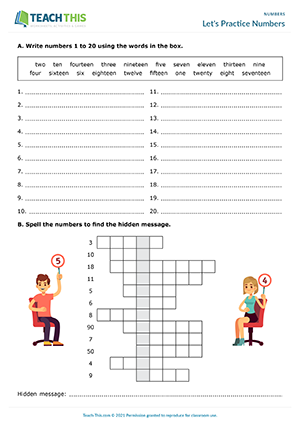## Numbers 1 to 20 Worksheet

### ESL Numbers Worksheet - Vocabulary Exercises: Forming Words, Matching, Sequencing, Sums, Word Search - Beginner (A1) - 20 minutes

This comprehensive numbers worksheet helps students learn and practice spelling numbers 1 to 20. Students begin by writing missing letters to make numbers and then matching the numbers to pictures. Students then write the next number in a set of sequences. Next, students complete sums. Lastly, students find the numbers from Exercise C in a word search.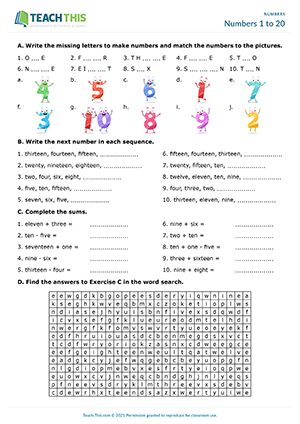## Sum it up

### ESL Numbers Crossword - Speaking Activity: Crossword, Doing Sums - Pair Work - Beginner (A1) - 20 minutes

In this engaging numbers crossword activity, students complete a number crossword by doing sums. In pairs, students take it in turns to ask their partner for a clue to one of the missing numbers on their crossword. Their partner then reads the sum that corresponds to that number and the other student does the sum. When the student has the answer, they spell the number. If the spelling is correct, the student writes the number on their crossword. If not, the student must try to spell the number again until they get it right.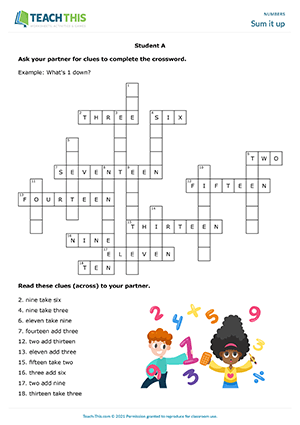## Can I check your phone number?

### ESL Phone Numbers Activity - Listening and Speaking: Checking, Correcting - Group Work - Elementary (A1-A2) - 20 minutes

In this useful saying phone numbers activity, students check and correct phone numbers. This activity is useful for practicing numbers 0 to 9. In groups, students assume the identity of the person whose name and phone number is at the top of their card. The other names and phone numbers on their card are the other students in their group, but each phone number is wrong. The students' task is to speak to each person in their group and correct their phone number. Students go around their group, speaking to each classmate in turn. When a student speaks to another classmate, they ask for their name and check the phone number next to the name on their card. Their classmate then gives the student the correct phone number. The student confirms the number back and corrects the phone number on their card. When the students have finished, they compare cards with a partner to make sure they have the same corrected phone numbers.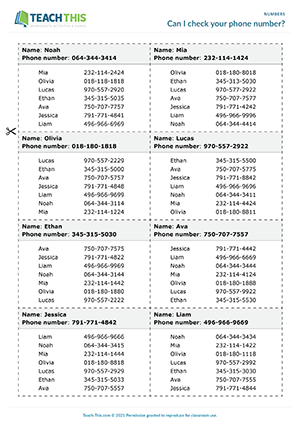## Mathematical Bingo

### ESL Numbers Game - Vocabulary: Doing Sums, Matching - Elementary (A1-A2) - 25 minutes

In this entertaining numbers game, students play bingo by completing sums and matching the answers to numbers on a bingo card. This game helps students practice numbers 0 to 50. Sums are called out at random from a caller's sheet. Students then do the sum. If a student has the answer on their bingo card, they cross the number off. When a student has crossed off all nine numbers, they shout 'bingo' and read out the numbers. If the numbers are correct, the student wins the round. Students play several rounds using a different bingo card each time.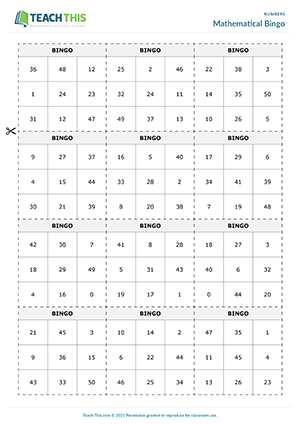## Hundreds Trivia

### ESL Large Numbers Activity and Quiz - Vocabulary: Running Dictation, Unscrambling, Quiz, Guessing - Group and Pair Work - Pre-intermediate (A2) - 35 minutes

Here is a fun numbers activity and trivia quiz to help students practice numbers between 100 and 1000. First, students take part in a running dictation. Student A is the writer and Student B is the reader. The readers run to 'Set A', read the first set of numbers, remember them, run back, and dictate them to their partner, who writes the numbers down. This continues until all ten sets of numbers have been dictated. Students then swap roles for 'Set B' and the process is repeated. The first pair to write all the number sets down correctly wins. In two groups, students then put words in the correct order to form trivia questions about numbers. Next, students pair up with someone new from the other group and take turns reading each trivia question along with two possible answers to their partner, who has to guess the correct answer. For each correct answer, students score one point. The student with the most points at the end of the quiz wins.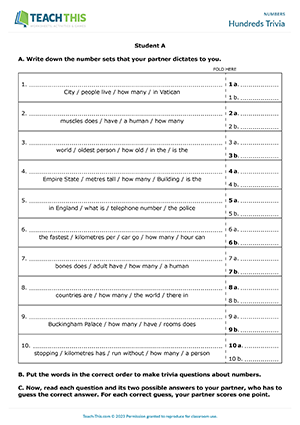## Mathematical Geniuses

### ESL Large Numbers Game - Vocabulary and Listening: Problem-solving, Guessing, Controlled Practice - Group Work - Pre-intermediate (A2) - 25 minutes

In this fast-paced numbers game, students race to calculate answers to mathematical problems. In groups, students take turns picking up a card and reading the mathematical problem on the card to the other students. The other students listen and then race to calculate the correct answer using paper to make their calculations. The first student to put up their hand and give the correct answer wins and keeps the card. If a student gives an incorrect answer, they are out of the round, and the other students continue trying to calculate the answer. If no one can guess the correct answer, the card is removed from the game. The student with the most cards at the end of the game wins.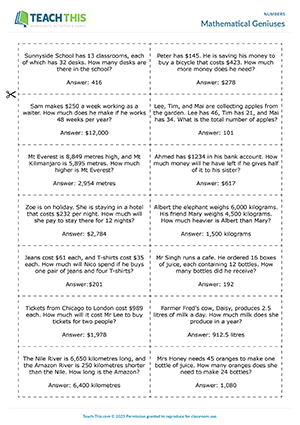## Numbers Stress Test

### ESL Numbers Game - Vocabulary: Listening, Pronunciation, Identifying - Group Work - Pre-intermediate (A2) - 15 minutes

In this amusing numbers game, students practice distinguishing between the sounds of -teen and -ty numbers. In groups of three, one student is the speaker, and the other two students are players. The speaker calls out a -teen or -ty number from one of the cards spread face-up on the table. The first player to touch the correct card scores a point. If the speaker mispronounces a number, a player can challenge them by asking the teacher to check the speaker's pronunciation. If the pronunciation is wrong, the player scores a point. The first player to score 12 points wins the round. Students then swap roles, and a new round begins. This process continues until all the students have been the speaker. The student who wins the most rounds is the winner.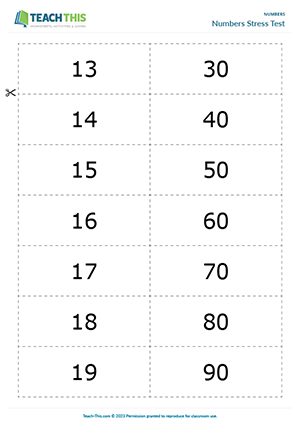## Confusing Numbers

### ESL Confusing Numbers Activity - Vocabulary and Pronunciation: Stressing Syllables, Matching - Group Work - Intermediate (B1) - 25 minutes

In this free confusing numbers activity, students practice saying large numbers ending with teen and ty. In groups, the student with 'start' on their card begins by saying the number indicated in the 'Say' column next to 'start'. The other students listen carefully and try to find the number in the 'Hear' column on their card. This can be quite challenging as the numbers have a very similar sound, e.g. 118 and 180. So, students need to pay particular attention to how they pronounce the numbers. If a student sees a matching number in the 'Hear' column, they say the corresponding number in the 'Say' column for the other students to recognise. The turn then passes from student to student until they reach the finish. When the students have finished, distribute the cards for Game B. The groups then repeat the activity with the new game cards.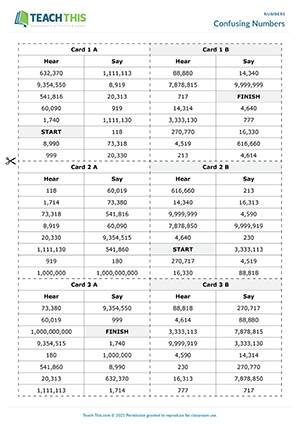## Numbers Quiz

### ESL Numbers Quiz Game - Listening and Speaking: Dictation, Listening for Detail, Matching - Pair Work - Intermediate (B1) - 30 minutes

In this productive numbers game, students dictate and write down large numbers and then use the numbers to complete a quiz. Each student memorizes a number on a card. Students then write numbers 1 to 16 on a piece of paper and go around the class telling each other their numbers. Students listen to their classmates' numbers and write them on the piece of paper. When the students have written down all 15 numbers, they sit down and add their number at the end. In pairs, students compare numbers with their partner. Next, pairs try to match the numbers with facts on a worksheet and write down their answers. Pairs score one point for each correct answer. The pair with the most points wins the game.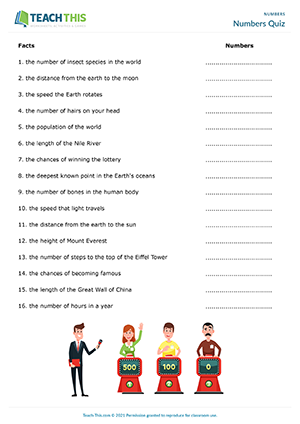## The Population Game

### ESL Large Numbers Game - Vocabulary and Speaking: Asking and Answering Questions, Guessing - Group Work - Intermediate (B1) - 30 minutes

In this memorable large numbers game, students guess the exact population of a country by asking Are there more/less than questions. In groups, students take it in turns to pick up a card and tell the group the name of the country on the card, e.g. Brunei. The group members then take turns asking a question to the student to determine the exact population of the country. They do this by asking Are there questions with more than and less than, e.g. 'Are there more than one million people living in Brunei?' The student with the card answers Yes, there are or No, there aren't, according to the number written on the card. If a group member receives a 'yes' answer, they ask another question. If the answer is 'no', play passes to the next student. This continues until a group member has established the exact population on their turn. When this happens, the student asks an Are there question about the exact population size, e.g. 'Are there 422,500 people living in Brunei?' That student wins the round and keeps the card. The student with the most cards at the end of the game wins.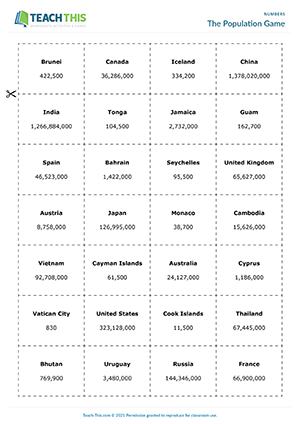## Fractional Facts

### ESL Fractions Game - Vocabulary: Matching, Guessing - Group and Pair Work - Upper-intermediate (B2) - 25 minutes

In this enjoyable fractions guessing game, students complete numerical facts by guessing fractions. In two groups, students begin by matching fractions to numerical facts and writing them down next to each sentence. Next, students pair up with someone from the other group and take turns reading the facts to their partner using the word 'blank' for the number in bold. After hearing the sentence, their partner chooses a fraction from a box on their worksheet that they think completes the fact. If the fraction is correct, the student puts a tick next to the fact. The student with the most correct guesses at the end of the game wins.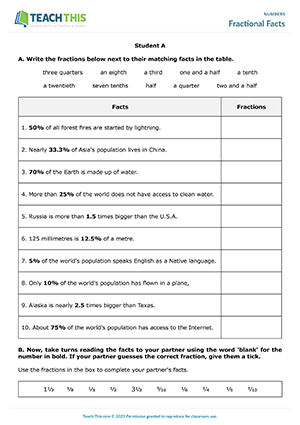## Fraction and Percentage Dominoes

### ESL Fractions and Percentages Game - Vocabulary: Matching - Group Work - Upper-intermediate (B2) - 20 minutes

In this handy fractions and percentages game, students play dominoes by matching numerical concepts expressed in different terms or symbols. The first player tries to match quantities of equal value by placing a domino down either before or after the domino on the table. If the player does this successfully, they read the fraction or percentage aloud. The next player then tries to put down one of their dominoes at either end of the domino chain and so on. The first player to get rid of all their dominoes wins the game.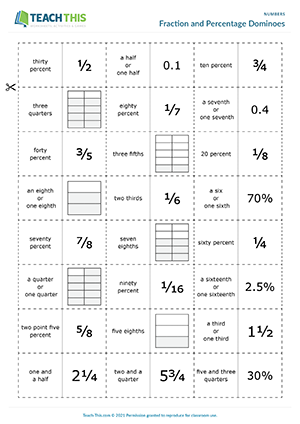## Talking Numbers

### ESL Maths Vocabulary Worksheet - Vocabulary Exercises: Matching, Gap-fill, Multiple Choice - Speaking Activity: Asking and Answering Questions - Pair Work - Upper-intermediate (B2) - 30 minutes

In this insightful numbers worksheet, students practice vocabulary related to maths. To begin, students match each maths noun to its definition. Students then use words in a box to complete maths-related sentences. Next, students underline the correct maths word to complete each sentence. After that, students use maths vocabulary from a box to complete trivia questions. Students then guess and tick what they think is the correct answer for each question. Finally, students ask and answer maths conversation questions with a partner, giving as much detail as possible.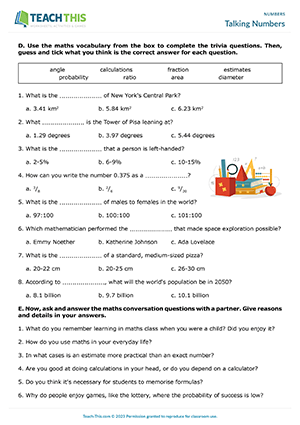Now Available!